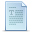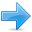# Constants

Constants are expressions with a fixed value.

### Literals

Literals are the most obvious kind of constants. They are used to express particular values within the source code of a program. We have already used some in previous chapters to give specific values to variables or to express messages we wanted our programs to print out, for example, when we wrote:

 ``1`` ``a = 5;``

The `5` in this piece of code was a literal constant.

Literal constants can be classified into: integer, floating-point, characters, strings, Boolean, pointers, and user-defined literals.

#### Integer Numerals

 ``123`` ``````1776 707 -``````

These are numerical constants that identify integer values. Notice that they are not enclosed in quotes or any other special character; they are a simple succession of digits representing a whole number in decimal base; for example, `1776` always represents the value one thousand seven hundred seventy-six.

In addition to decimal numbers (those that most of us use every day), C++ allows the use of octal numbers (base 8) and hexadecimal numbers (base 16) as literal constants. For octal literals, the digits are preceded with a `0` (zero) character. And for hexadecimal, they are preceded by the characters `0x` (zero, x). For example, the following literal constants are all equivalent to each other:

 ``123`` ``````75 // decimal 0113 // octal 0x4b // hexadecimal ``````

All of these represent the same number: 75 (seventy-five) expressed as a base-10 numeral, octal numeral and hexadecimal numeral, respectively.

These literal constants have a type, just like variables. By default, integer literals are of type `int`. However, certain suffixes may be appended to an integer literal to specify a different integer type:

SuffixType modifier
`u` or `U``unsigned`
`l` or `L``long`
`ll` or `LL``long long`

Unsigned may be combined with any of the other two in any order to form `unsigned long` or `unsigned long long`.

For example:

 ``12345`` ``````75 // int 75u // unsigned int 75l // long 75ul // unsigned long 75lu // unsigned long ``````

In all the cases above, the suffix can be specified using either upper or lowercase letters.

#### Floating Point Numerals

They express real values, with decimals and/or exponents. They can include either a decimal point, an `e` character (that expresses "by ten at the Xth height", where X is an integer value that follows the `e` character), or both a decimal point and an `e` character:

 ``1234`` ``````3.14159 // 3.14159 6.02e23 // 6.02 x 10^23 1.6e-19 // 1.6 x 10^-19 3.0 // 3.0 ``````

These are four valid numbers with decimals expressed in C++. The first number is PI, the second one is the number of Avogadro, the third is the electric charge of an electron (an extremely small number) -all of them approximated-, and the last one is the number three expressed as a floating-point numeric literal.

The default type for floating-point literals is `double`. Floating-point literals of type `float` or `long double` can be specified by adding one of the following suffixes:

SuffixType
`f` or `F``float`
`l` or `L``long double`

For example:

 ``12`` ``````3.14159L // long double 6.02e23f // float ``````

Any of the letters that can be part of a floating-point numerical constant (`e`, `f`, `l`) can be written using either lower or uppercase letters with no difference in meaning.

#### Character and string literals

Character and string literals are enclosed in quotes:

 ``1234`` ``````'z' 'p' "Hello world" "How do you do?"``````

The first two expressions represent single-character literals, and the following two represent string literals composed of several characters. Notice that to represent a single character, we enclose it between single quotes (`'`), and to express a string (which generally consists of more than one character), we enclose the characters between double quotes (`"`).

Both single-character and string literals require quotation marks surrounding them to distinguish them from possible variable identifiers or reserved keywords. Notice the difference between these two expressions:

``` x 'x' ```
Here, `x` alone would refer to an identifier, such as the name of a variable or a compound type, whereas `'x'` (enclosed within single quotation marks) would refer to the character literal `'x'` (the character that represents a lowercase x letter).

Character and string literals can also represent special characters that are difficult or impossible to express otherwise in the source code of a program, like newline (`\n`) or tab (`\t`). These special characters are all of them preceded by a backslash character (`\`).

Here you have a list of the single character escape codes:

Escape codeDescription
`\n`newline
`\r`carriage return
`\t`tab
`\v`vertical tab
`\b`backspace
`\f`form feed (page feed)
`\a`alert (beep)
`\'`single quote (`'`)
`\"`double quote (`"`)
`\?`question mark (`?`)
`\\`backslash (`\`)

For example:

``` '\n' '\t' "Left \t Right" "one\ntwo\nthree" ```
Internally, computers represent characters as numerical codes: most typically, they use one extension of the ASCII character encoding system (see ASCII code for more info). Characters can also be represented in literals using its numerical code by writing a backslash character (`\`) followed by the code expressed as an octal (base-8) or hexadecimal (base-16) number. For an octal value, the backslash is followed directly by the digits; while for hexadecimal, an `x` character is inserted between the backslash and the hexadecimal digits themselves (for example: `\x20` or `\x4A`).

Several string literals can be concatenated to form a single string literal simply by separating them by one or more blank spaces, including tabs, newlines, and other valid blank characters. For example:

 ``12`` ``````"this forms" "a single" " string " "of characters"``````

The above is a string literal equivalent to:

 ``1`` ``"this formsa single string of characters"``

Note how spaces within the quotes are part of the literal, while those outside them are not.

Some programmers also use a trick to include long string literals in multiple lines: In C++, a backslash (`\`) at the end of line is considered a line-continuation character that merges both that line and the next into a single line. Therefore the following code:

 ``12`` ``````x = "string expressed in \ two lines"``````

is equivalent to:

 ``1`` ``x = "string expressed in two lines"``

All the character literals and string literals described above are made of characters of type `char`. A different character type can be specified by using one of the following prefixes:

PrefixCharacter type
`u``char16_t`
`U``char32_t`
`L``wchar_t`

Note that, unlike type suffixes for integer literals, these prefixes are case sensitive: lowercase for `char16_t` and uppercase for `char32_t` and `wchar_t`.

For string literals, apart from the above `u`, `U`, and `L`, two additional prefixes exist:

PrefixDescription
`u8`The string literal is encoded in the executable using UTF-8
`R`The string literal is a raw string

In raw strings, backslashes and single and double quotes are all valid characters; the content of the literal is delimited by an initial `R"sequence(` and a final `)sequence"`, where `sequence` is any sequence of characters (including an empty sequence). The content of the string is what lies inside the parenthesis, ignoring the delimiting sequence itself. For example:

 ``12`` ``````R"(string with \backslash)" R"&%\$(string with \backslash)&%\$"``````

Both strings above are equivalent to `"string with \\backslash"`. The `R` prefix can be combined with any other prefixes, such as `u`, `L` or `u8`.

#### Other literals

Three keyword literals exist in C++: `true`, `false` and `nullptr`:
• `true` and `false` are the two possible values for variables of type `bool`.
• `nullptr` is the null pointer value.

 ``123`` ``````bool foo = true; bool bar = false; int* p = nullptr;``````

### Typed constant expressions

Sometimes, it is just convenient to give a name to a constant value:

 ``12`` ``````const double pi = 3.1415926; const char tab = '\t';``````

We can then use these names instead of the literals they were defined to:

 ``12345678910111213141516171819202122232425262728293031`` ``````#include using namespace std; const double pi = 3.14159; const char newline = '\n'; int main () { double r=5.0; // radius double circle; circle = 2 * pi * r; cout << circle; cout << newline; }`````` `31.4159`

### Preprocessor definitions (#define)

Another mechanism to name constant values is the use of preprocessor definitions. They have the following form:

`#define identifier replacement`

After this directive, any occurrence of `identifier` in the code is interpreted as `replacement`, where replacement is any sequence of characters (until the end of the line). This replacement is performed by the preprocessor, and happens before the program is compiled, thus causing a sort of blind replacement: the validity of the types or syntax involved is not checked in any way.

For example:
 ``12345678910111213141516171819202122232425262728293031`` ``````#include using namespace std; #define PI 3.14159 #define NEWLINE '\n' int main () { double r=5.0; // radius double circle; circle = 2 * PI * r; cout << circle; cout << NEWLINE; }`````` `31.4159`

Note that the `#define` lines are preprocessor directives, and as such are single-line instructions that -unlike C++ statements- do not require semicolons (;) at the end; the directive extends automatically until the end of the line. If a semicolon is included in the line, it is part of the replacement sequence and is also included in all replaced occurrences.Previous:Variables and typesIndexNext:Operators